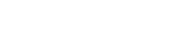# math.cosh

`FLOATmath.coshFLOATx`

Available inall subroutines.

Computes the hyperbolic cosine of its argument `x`.

## Parameters

`x` - Floating point value representing a hyperbolic angle.

## Return Value

Upon successful completion, this function returns the hyperbolic cosine of `x`.

If `x` is `math.NAN`, a NaN will be returned.

If `x` is ±0, the value 1.0 will be returned.

If `x` is `math.POS_INFINITY` or `math.NEG_INFINITY`, `math.POS_INFINITY` will be returned.

If the result would cause an overflow, a range error occurs and `math.cosh()` will return the value of the macro `math.POS_HUGE_VAL`.

## Errors

If the result would cause an overflow, then `fastly.error` will be set to `ERANGE`.

## Example

``````declare local var.fo FLOAT;
set var.fo = math.cosh(0);``````

BETA